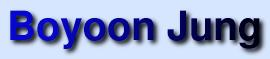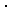Boyoon Jung's Library Manual

# bj::VeryFastEMCluster Class Reference [EM-based Clustering]

A very fast EM-based clustering algorithm using multiresolution kd-trees. More...

`#include <em_cluster.h>`

Collaboration diagram for bj::VeryFastEMCluster:[legend]
List of all members.

## Public Member Functions

VeryFastEMCluster (int dimension, int max_data, gsl_vector *min=0, gsl_vector *max=0, int max_clusters=10, int max_iteration=100)
A constructor.
~VeryFastEMCluster (void)
A destructor.
void initialize (double *pi=0, gsl_vector **mean=0, gsl_matrix **cov=0)
Initialize EM cluster.
virtual int cluster (gsl_vector **data, int size, double mbw=-10)
Cluster data points using gaussian mixture.
int numClusters (void)
Return the number clusters.
double weight (int c)
Return the weights of a specific cluster.
gsl_vector * mean (int c)
Return the mean vector of a specific cluster.
gsl_matrix * covariance (int c)
Return the covariance matrix of a specific cluster.
MRKDTreetree (void)
Return the multiresolution KD-tree.
void setTerminationParams (double prt=0.0, double pat=1.e-8, double mrt=0.0, double mat=1.e-5, double crt=0.0, double cat=1.e-5)
Set the tolerance values for convergence test.

## Static Public Member Functions

void cov2ellipse (gsl_matrix *cov, double &w, double &h, double &a)
Convert a covariance matrix to an ellipsoidal parameters.

## Detailed Description

A very fast EM-based clustering algorithm using multiresolution kd-trees.

VeryFastEMCluster class implements the EM-based clustering algorithm using multiresolution kd-trees (presented by Moore, 1999).

The current version does not implement the second stage (pruning to save more time without introducing significant error).

## Constructor & Destructor Documentation

 bj::VeryFastEMCluster::VeryFastEMCluster ( int dimension, int max_data, gsl_vector * min = `0`, gsl_vector * max = `0`, int max_clusters = `10`, int max_iteration = `100` )

A constructor.

Parameters:
 dimension The dimension of data points. max_data The maximum number of data points. min The lower bound of the data space. max The upper bound of the data space. max_clusters The maximum number of clusters. max_iteration The upper bound of iteration.

 bj::VeryFastEMCluster::~VeryFastEMCluster ( void )
 A destructor.

## Member Function Documentation

 virtual int bj::VeryFastEMCluster::cluster ( gsl_vector ** data, int size, double mbw = `-10` ) ` [virtual]`
 Cluster data points using gaussian mixture. Returns:The number of iteration.

 void bj::VeryFastEMCluster::cov2ellipse ( gsl_matrix * cov, double & w, double & h, double & a ) ` [inline, static]`

Convert a covariance matrix to an ellipsoidal parameters.

Parameters:
 cov A covariance matrix. [out] w The width of an ellipsoidal. [out] h The height of an ellipsoidal. [out] a The angle (in radians) of an ellipsoidal.

 gsl_matrix* bj::VeryFastEMCluster::covariance ( int c ) ` [inline]`
 Return the covariance matrix of a specific cluster.

 void bj::VeryFastEMCluster::initialize ( double * pi = `0`, gsl_vector ** mean = `0`, gsl_matrix ** cov = `0` )
 Initialize EM cluster.

 gsl_vector* bj::VeryFastEMCluster::mean ( int c ) ` [inline]`
 Return the mean vector of a specific cluster.

 int bj::VeryFastEMCluster::numClusters ( void ) ` [inline]`
 Return the number clusters.

 void bj::VeryFastEMCluster::setTerminationParams ( double prt = `0.0`, double pat = `1.e-8`, double mrt = `0.0`, double mat = `1.e-5`, double crt = `0.0`, double cat = `1.e-5` ) ` [inline]`
 Set the tolerance values for convergence test.

 MRKDTree* bj::VeryFastEMCluster::tree ( void ) ` [inline]`
 Return the multiresolution KD-tree.

 double bj::VeryFastEMCluster::weight ( int c ) ` [inline]`
 Return the weights of a specific cluster.

The documentation for this class was generated from the following file:
ResearchProjectsPublications

ToolsBjLib ManualPlayer/StagePyPlayerMicroStrain IMUGPS/DGPSParticle Filter

PersonalWelcomeCurriculum VitaeResumeContact

Outside LinksUSCUSC CS DeptCRESRobotics LabRESL